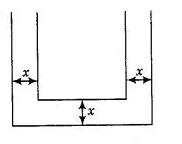# How many cubic centimetres of iron is required

Question:

How many cubic centimetres of iron is required to construct an open box whose external dimensions are 36 cm, 25 cm and 16.5 cm provided the

thickness of the iron is 1.5 cm. If one cubic centimetre of iron weights 7.5 g, then find the weight of the box.

Solution:

Let the length $(l)$, breadth (b) and height ( $h$ ) be the external dimension of an open box and thickness be $x$.

Given that,external length of an open box $(l)=36 \mathrm{~cm}$

external breadth of an open box $(b)=25 \mathrm{~cm}$

and external height of an open box $(h)=16.5 \mathrm{~cm}$

$\therefore$ External volume of an open box $=l b h$

$=36 \times 25 \times 16.5$

$=14850 \mathrm{~cm}^{3}$

Since, the thickness of the iron $(x)=1.5 \mathrm{~cm}$

So, internal length of an open box $(\zeta)=l-2 x$

$=36 \times 2 \times 1.5$

$=36-3=33 \mathrm{~cm}$

Therefore, internal breadth of an open box $\left(b_{2}\right)=b-2 x$

$=25-2 \times 1.5=25-3=22 \mathrm{~cm}$

and internal height of an open box $\left(h_{2}\right)=(h-x)$

$=16.5-1.5=15 \mathrm{~cm}$

So, internal volume of an open box $=(l-2 x) \cdot(b-2 x) \cdot(h-x)$

$=33 \times 22 \times 15=10890 \mathrm{~cm}^{3}$

Therefore required iron to construct an open box

$=$ External volume of an open box - Internal volume of an open box

$=14850-10890=3960 \mathrm{~cm}^{3}$

Hence, required iron to construct an open box is $3960 \mathrm{~cm}^{3}$.

Given that, $1 \mathrm{~cm}^{3}$ of iron weights $=7.5 \mathrm{~g}=\frac{7.5}{1000} \mathrm{~kg}=0.0075 \mathrm{~kg}$

$\therefore \quad 3960 \mathrm{~cm}^{3}$ of iron weights $=3960 \times 0.0075=29.7 \mathrm{~kg}$